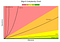# Big O Notation in Programming

As a programmer we have seen the Big O notation many times, yet I noticed this is one concept that a lot of beginner programmers have a hard time understanding. It’s not the concept is hard to understand, it basically describe the performance or the complexity of an algorithm. Most people tend to overthink it and in their head they believe it’s an extremely complicated mathematic thing that you need to major in math to understand it.

# What is the Big O Notation in an Algorithm?

The big O notation is basically a way to tell how long it takes for a certain code to run in respect to the amount of elements/items. There are many different notations but the few basic notations are O(1), O(n), O(n²), O(2^n) and O(log N). We usually use this to determine how effective an algorithm is if we are dealing with an large amount of data. Here is a basic cheat sheet, the big O complexity chart.Found from bigocheatsheet.com

## O(1)

O(1) is known as constant complexity. It’s an algorithm that will always run in the same amount of time, regardless of the amount of input data. Something like if you are checking if the first element is a certain number or not.

## O(N)

O(N) is known as linear complexity. The amount of items will grow linearly in proportion to the size of the input data. An example will be having a loop checking every single element to determine if the item exist within the array.

## O(N²)

O(N²) is known as quadratic complexity. The performance of the algorithm is proportional to the square of the input data. This is commonly found in algorithms that have nested loops over the data set. If the nested loops gets deeper and deeper, it will result in O(N³), O(N⁴) and so on.

## O(2^N)

O(2^N) is known as exponential complexity. The performance of the algorithm doubles with every new addition to the data set. An example of this is the Fibonacci numbers.

## O(log N)

O(log N) is known as logarithmic complexity. The performance of this algorithm will produce a growth curve that is really bad early on, but as the data set gets bigger, it slowly flattens out to be similar to O(N). An example of this is Binary Search, and you can find a blog I did on Binary Search here.

Just a student at flatiron

## More from Fanzhong Zeng

Just a student at flatiron